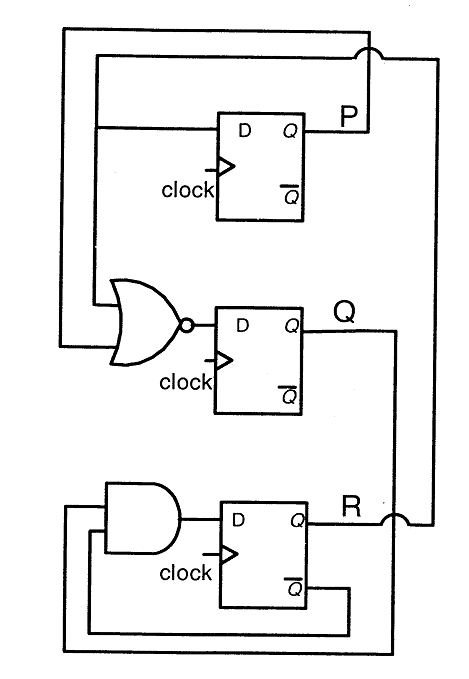Skip to content
Related Articles
GATE | GATE CS 2011 | Question 65
• Last Updated : 19 Nov, 2018

Consider the following circuit involving three D-type flip-flops used in a certain type of counter configuration.If at some instance prior to the occurrence of the clock edge, P, Q and R have a value 0, 1 and 0 respectively, what shall be the value of PQR after the clock edge?
(A) 000
(B) 001
(C) 010
(D) 011

Answer: (D)

Explanation: P’ = R
Q’ = (P + R)’
R’ = QR’ Given that (P, Q, R) = (0, 1, 0), next state P’, Q’, R’ = 0, 1, 1

———————————————————————————————–

D flip flop truth table

 D Q(t+1) 0 0 1 1

Initially (p,q,r) =(0,1,0)
D for p=R
D for q=NOT(p xor r)
D for r= (not)r.q
So Q(t+1) for(p,q,r)
p=>r=0 so p=0
q=> NOT(p xor r) => 1      so q=1
r=>(not)r.q => 1         so r=1
(p, q, r) = (0, 1, 1)

Alternative approach –
Truth table of a D Flip-Flop-By looking at the circuit diagram, it is clear that the boolean expressions of P, Q, and R are-
Here the subscript t refers to the current clock cycle, and the subscript (t+1) refers to the next clock cycle.This explanation is provided by Chirag Manwani.

Quiz of this Question

Attention reader! Don’t stop learning now. Learn all GATE CS concepts with Free Live Classes on our youtube channel.

My Personal Notes arrow_drop_up